﻿ 电器对低压电力线载波通信信道特性的影响文章快速检索 高级检索
 重庆邮电大学学报(自然科学版)2020, Vol. 32Issue (3): 411-418  DOI: 10.3979/j.issn.1673-825X.2020.03.0100

### 引用本文WANG Yi, JIA Rui, LIANG Xing, ZHENG Ke, YE Jun, LI Songnong. Influence of electrical appliance on the channel characteristics of low voltage power line carrier communication[J]. Journal of Chongqing University of Posts and Telecommunications (Natural Science Edition), 2020, 32(3): 411-418.   DOI: 10.3979/j.issn.1673-825X.2020.03.010.### Foundation item

The China Postdoctoral Science Foundation Funded Project (2015T80961);The Natural Science Foundation of Chongqing Science and Technology Commission (cstc2016jcyjA0214)

.

### 文章历史

1. 重庆邮电大学 通信与信息工程学院, 重庆 400065;
2. 国网重庆市电力公司电力科学研究院, 重庆 400014;
3. 国网重庆市电力公司博士后科研工作站, 重庆 400014

Influence of electrical appliance on the channel characteristics of low voltage power line carrier communication
WANG Yi1,2,3, JIA Rui1, LIANG Xing2 , ZHENG Ke2 , YE Jun2 , LI Songnong2
1. School of Communication and Information Engineering, Chongqing University of Posts and Telecommunications, Chongqing 400065, P. R. China;
2. Chongqing Electric Power Research Institute, Chongqing 400014, P. R. China;
3. Postdoctoral Workstation of the Chongqing Electric Power Corporation, Chongqing 400014, P. R. China
Abstract: In order to study the impact of electrical appliance on the characteristics of low-voltage power line channel, this paper presents the two-dimensional time and frequency electrical appliance impedance measurement method based on a vector network analyzer. Through this method, the impedance characteristics of all kinds of electrical appliance are measured under different working conditions. Then, the channel characteristics of a typical power line channel scenario connecting to different types of electrical appliance loads are theoretically analyzed in combination with bottom-up power line channel modeling. According to the measurement, the same load of electrical appliance in different spatial locations affect the power line channels differently. The influence of different electrical appliance on the characteristics of power line channels is different when the load of electrical appliance is connected to the same load node. In addition, the time-varying load causes the power line channels accessing the load to present time-varying characteristics. Theoretical analysis and test results show that load impedance of electrical appliance with time-frequency changes is one of the key factors that affect the channel characteristics of low-voltage power line carrier communication.
Keywords: power line carrier communication    electrical appliance    load impedance    impedance measurement    channel characteristics
0 引言

1 电力线信道特性分析 1.1 衰减特性

 ${Z_c} = \sqrt {\frac{{R + {\rm{j}}\omega L}}{{G + {\rm{j}}\omega C}}}$ (1)
 $\gamma = \sqrt {(R + {\rm{j}}\omega L)(G + {\rm{j}}\omega C)} = \alpha + {\rm{j}}\beta$ (2)

(1)—(2)式中：ω为传输信号角频率；RLCG分别表示该电力线传输线在单位长度上的电阻、电感、电容和电导；α为衰减常数，α=Re(γ)；β为波相速，β=Im(γ)。为便于工程上对电力传输线建模，能够通过下面的参数模型对复传播常数进行描述为

 ${\alpha (f) = ({a_0} + {a_1}{f^K})}$ (3)
 ${\beta (f) = \frac{{2\pi f}}{{{V_p}}}}$ (4)

(3)—(4)式中：a0a1K为常量；Vp为信号在电力传输线传播速度，与导线间绝缘材料介电常数有关；f为传输信号频率。

1.2 阻抗特性

2 自下而上的信道建模方法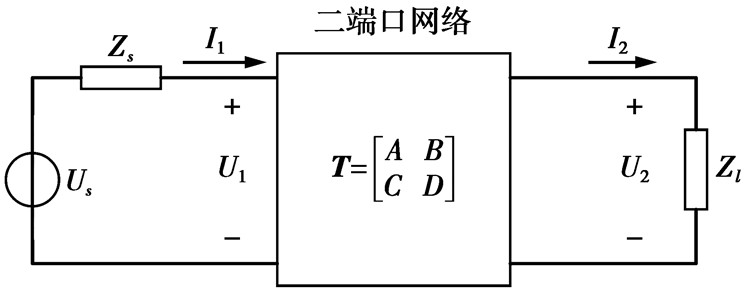图 1 二端口网络模型 Fig.1 Two-port network model
 $\left[ {\begin{array}{*{20}{c}} {{U_1}}\\ {{I_1}} \end{array}} \right] = \left[ {\begin{array}{*{20}{c}} A&B\\ C&D \end{array}} \right]\left[ {\begin{array}{*{20}{c}} {{U_2}}\\ {{I_2}} \end{array}} \right]$ (5)

(5) 式中复系数ABCD为频率f的函数，表征了二端口网络的电气特性。ABCD可以表示为

 $\begin{array}{l} A = \frac{{{U_1}}}{{{U_2}}}|({I_2} = 0){\kern 1pt} {\kern 1pt} {\kern 1pt} {\kern 1pt} {\kern 1pt} {\kern 1pt} {\kern 1pt} {\kern 1pt} {\kern 1pt} {\kern 1pt} {\kern 1pt} {\kern 1pt} B = \frac{{{U_1}}}{{{I_2}}}|({U_2} = 0)\\ C = \frac{{{I_1}}}{{{U_2}}}|({I_2} = 0){\kern 1pt} {\kern 1pt} {\kern 1pt} {\kern 1pt} {\kern 1pt} {\kern 1pt} {\kern 1pt} {\kern 1pt} {\kern 1pt} {\kern 1pt} {\kern 1pt} D = \frac{{{I_1}}}{{{I_2}}}|({U_2} = 0) \end{array}$ (6)

 ${Z_{{\rm{in}}}}(f) = \frac{{A{Z_l} + B}}{{C{Z_l} + D}}$ (7)
 $H(f) = \frac{{{U_2}}}{{{U_s}}} = \frac{{{Z_l}}}{{A{Z_l} + B + C{Z_s}{Z_l} + D{Z_s}}}$ (8)

 ${H_{s, t}}(f) = \frac{{{U_t}}}{{{U_s}}} = \frac{{{Z_t}}}{{{A_{s, t}}{Z_t} + {B_{s, t}} + {C_{s, t}}{Z_s}{Z_t} + {D_{s, t}}{Z_s}}}$ (9)

(9) 式中：Zs为发送端源阻抗；Zt为接收端负载阻抗。

3 电器负载阻抗特性 3.1 测量方法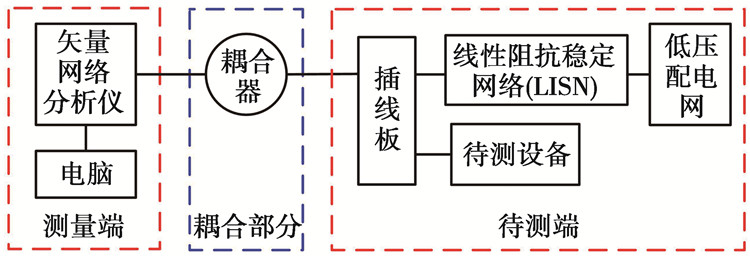图 2 电器阻抗测量框图 Fig.2 Electrical appliances impedance measurement block diagram

 ${Z_2} = \frac{{1 + {r_2}}}{{1 - {r_2}}} \cdot {Z_0}$ (10)

(10) 式中，Z2为耦合器与被测端结点处的输入阻抗。用耦合器与测量端的反射系数r1表示r2时(s为耦合器的散射参数)得到

 ${r_2} = \frac{{{r_1} - {s_{11}}}}{{{s_{12}} \cdot {s_{21}} + {s_{22}} \cdot ({r_1} - {s_{12}})}}$ (11)

 ${Z_L} = \frac{{[(1 + {r_1}) \cdot {Z_0} - (1 - {r_1}) \cdot {Z_2}] \cdot {Z_0}}}{{(1 - {r_2}) \cdot ({Z_0} + {Z_2}) - (1 + {r_2}) \cdot {Z_2}}}$ (12)

 ${Z_{{\rm{Load}}}} = \frac{{{Z_{{\rm{LISN}}}}{Z_{{\rm{parallel}}}}}}{{{Z_{{\rm{LISN}}}} - {Z_{{\rm{parallel}}}}}}$ (13)
3.2 测量内容

3.3 测量结果及分析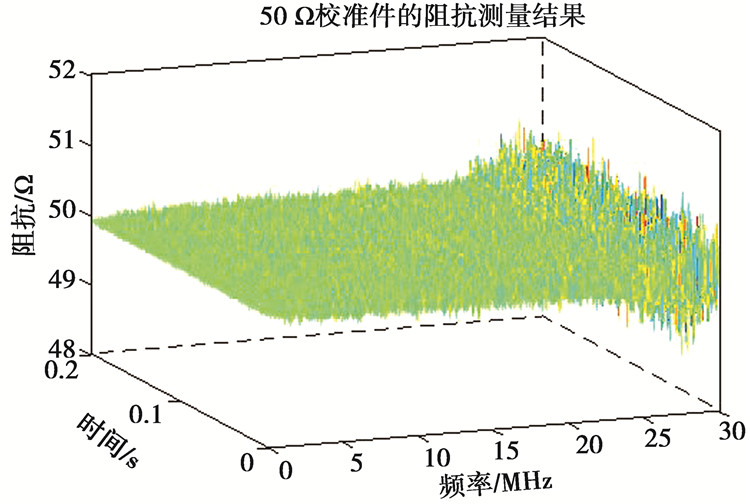图 3 50 Ω校准件的阻抗测量结果 Fig.3 Impedance measurement of 50 ohm calibration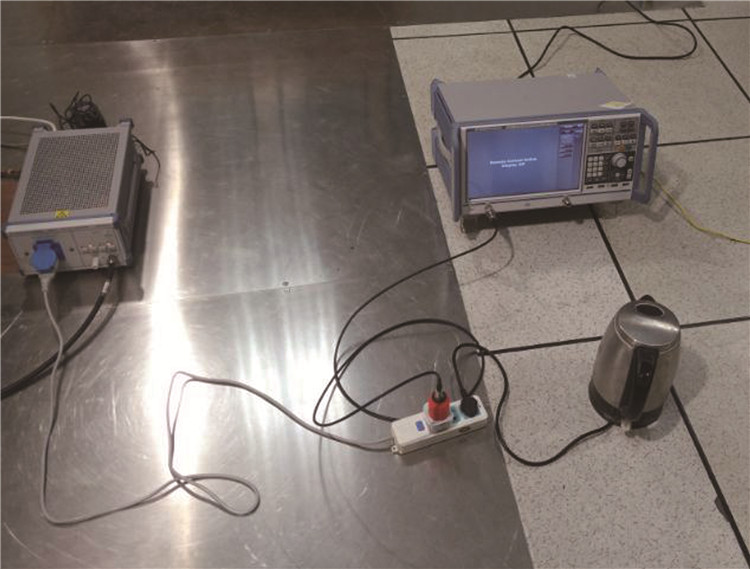图 4 电器阻抗测量的现场照片 Fig.4 Live photo of electrical appliances impedance measurement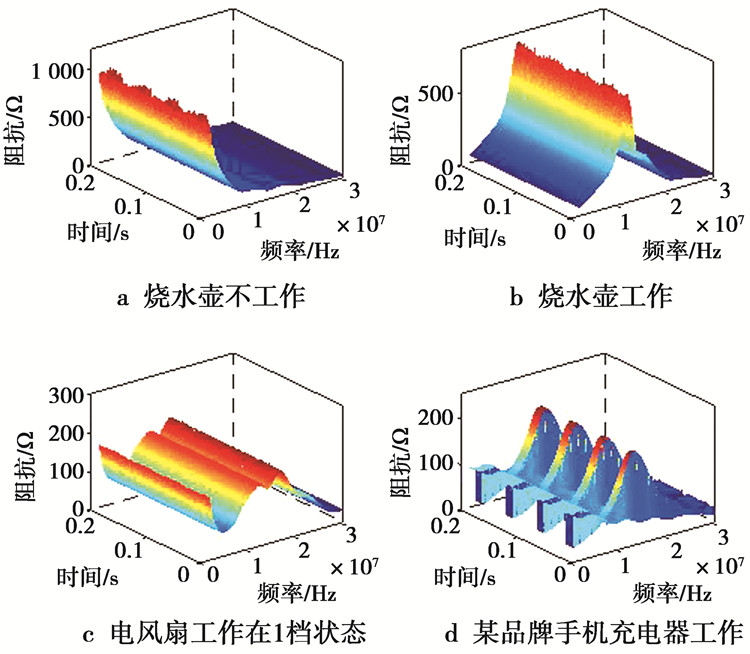图 5 电器阻抗测量结果 Fig.5 Electrical appliances impedance measurement results

4 电器对PLC信道特性的影响 4.1 理论分析方法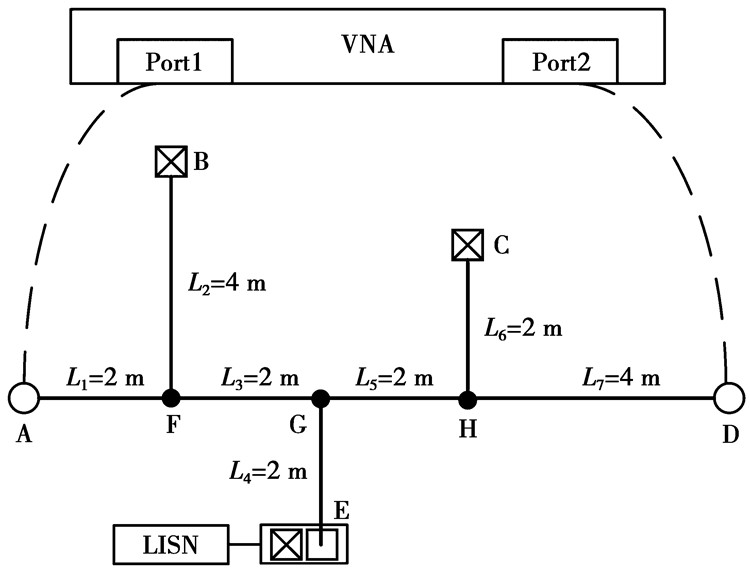图 6 测试场景 Fig.6 Test scenario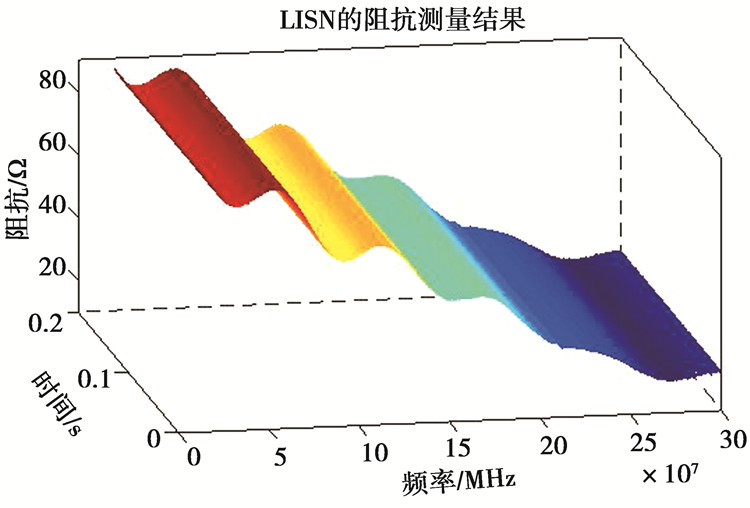图 7 LISN的阻抗测量结果 Fig.7 Impedance measurement of LISN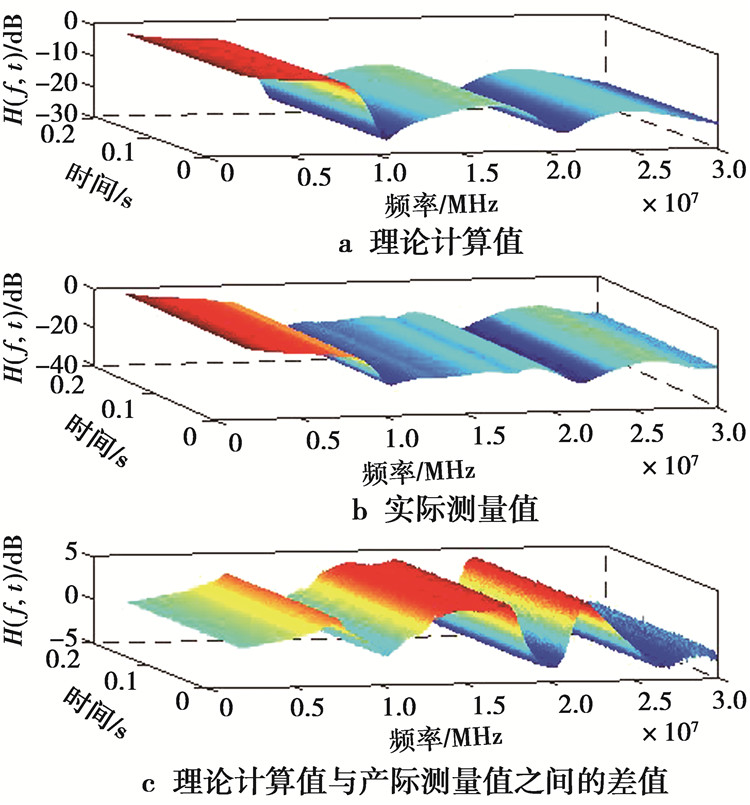图 8 理论计算值与实测数据的对比 Fig.8 Comparison between theoretical calculation and measured data
4.2 负载空间位置分布的影响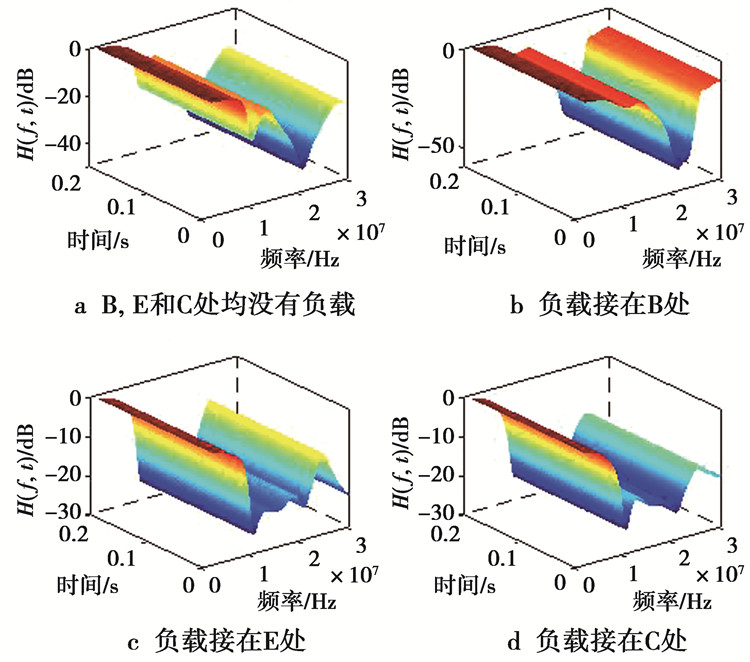图 9 相同负载接入不同位置时的电力线信道传输函数 Fig.9 Power line channel transfer function when the same load is connected to different positions

4.3 不同电器负载的影响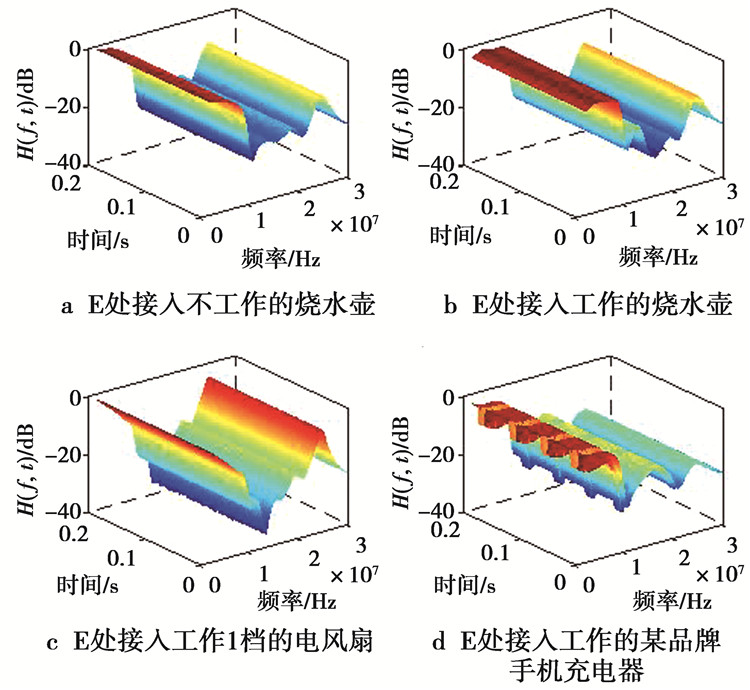图 10 接入不同负载时电力线信道传输函数 Fig.10 Power line channel transfer functions for different loads

5 结语

  ANASTASIADOU D, ANTONAKOPOULOS T. Multipath characterization of indoor power-line networks[J]. IEEE Transactions on Power Delivery, 2005, 20(1): 90-99. DOI:10.1109/TPWRD.2004.832373  马强, 陈启美, 李勃. 跻身未来的电力线通信(二)电力线信道分析及模型[J]. 电力系统自动化, 2003, 27(4): 72-76. MA Q, CHEN Q M, LI B. Predictive schemes for future power line communication part two PLC channel analysis and model[J]. Automation of Electric System, 2003, 27(4): 72-76. DOI:10.3321/j.issn:1000-1026.2003.04.017  高锋, 董亚波. 低压电力线载波通信中信号传输特性分析[J]. 电力系统自动化, 2000, 24(7): 36-40. GAO F, DONG Y B. Signal transmission characteristic analysis in low voltage power line carrier communication[J]. Automation of Electric System, 2000, 24(7): 36-40. DOI:10.3321/j.issn:1000-1026.2000.07.009  刘雪艳, 张强, 李战明, 等. 面向智能电网通信系统的数据聚合和访问控制方法[J]. 电力系统自动化, 2016, 40(14): 135-144. LIU X Y, ZHANG Q, LI Z M, et al. Data aggregation and access control method for smart grid communication system[J]. Automation of Electric Power Systems, 2016, 40(14): 135-144. DOI:10.7500/AEPS20151012002  李存斌, 李小鹏, 田世明, 等. 能源互联网电力信息深度融合风险传递:挑战与展望[J]. 电力系统自动化, 2017, 41(11): 17-25. LI C B, LI X P, TIAN S M, et al. Challenges and Prospects of risk transmission in deep fusion of electric power and information for energy internet[J]. Automation of Electric Power Systems, 2017, 41(11): 17-25. DOI:10.7500/AEPS20160927014  ZIMMERMANN M, DOSTERT K. A multipath model for the powerline channel[J]. IEEE Transactions on Communications, 2002, 50(4): 553-559. DOI:10.1109/26.996069  DEVELI L, KABALCI Y, BASTURK A. Artificial bee colony optimization for modelling of indoor PLC channels:A case study from Turkey[J]. Electric Power Systems Research, 2015(127): 73-79.  张旭辉, 林海军, 刘明珠, 等. 基于蚁群粒子群优化的卡尔曼滤波算法模型参数辨识[J]. 电力系统自动化, 2014, 38(4): 44-50. ZHANG X H, LIN H J, LIU M Z, et al. Model identification of UKF algorithm based on ACO-PSO[J]. Auto-mation of Electric Power Systems, 2014, 38(4): 44-50.  BANWELL T, GALLI S. A novel approach to the modeling of the indoor power line channel part i:circuit analysis and companion model[J]. IEEE Transactions on Power Delivery, 2005, 20(2): 655-663. DOI:10.1109/TPWRD.2005.844326  GALLI S, BANWELL T. A novel approach to the modeling of the indoor power line channel-Part II:transfer function and its properties[J]. IEEE Transactions on Power Delivery, 2005, 20(3): 1869-1878. DOI:10.1109/TPWRD.2005.848732  王艳, 王东, 赵洪山. 基于支路追加法的配电网混联线路电力线载波通信信道建模[J]. 电力系统自动化, 2016, 40(23): 56-60. WANG Y, WANG D, ZHAO H S. Power line carrier communication channel modeling of hybrid lines in distribution network based on branch additional method[J]. Automation of Electric Power Systems, 2016, 40(23): 56-60. DOI:10.7500/AEPS20160307006  蔡伟, 乐健, 刘开培, 等. 基于信息节点的智能配电网中压电力线载波通信信道建模方法[J]. 中国电机工程学报, 2012, 32(28): 150-156. CAI W, LE J, LIU K P, et al. A channel modeling technology for the medium-voltage power-line carrier in smart power distribution grid[J]. Proceedings of the CSEE, 2012, 32(28): 150-156.  TONELLO A M, VERSOLATTO F. Bottom-up statistical PLC channel modeling-part i:random topology model and efficient transfer function computation[J]. IEEE Transactions on Power Delivery, 2011, 26(2): 891-898. DOI:10.1109/TPWRD.2010.2096518  王毅, 邓子乔, 温慧安, 等. 基于多节点的低压宽带电力线信道建模方法研究[J]. 电力系统保护与控制, 2018, 46(3): 18-24. WANG Y, DENG Z Q, WEN H A, et al. The study on multi-node broadband power line channel modeling[J]. Power System Protection and Control, 2018, 46(3): 18-24.  张洪欣, 章学猛, 台新. 电力线信道噪声分析及建模研究[J]. 重庆邮电大学学报(自然科学版), 2013, 25(5): 593-598. ZHANG H X, ZHANG X M, TAI X. Noise analysis and modeling research in power line channel[J]. Journal of Chongqing University of Posts and Telecommunications(Natural Science Edition), 2013, 25(5): 593-598.  ANAATORY J, THEETHAYI N, THOTTAPPILLIL R, et al. The influence of load impedance, line length, and branches on underground cable power-line communications (PLC) systems[J]. IEEE Transactions on Power Delivery, 2007, 23(1): 180-187.  付科源, 郑涛, 张保会, 等. 电力线载波通信中电网结构参数对信道容量的影响[J]. 电力系统自动化, 2015, 39(13): 94-100. FU K Y, ZHENG T, ZHANG B H, et al. Effects of power network parameters on channel capacity in power line carrier communication[J]. Automation of Electric Power Systems, 2015, 39(13): 94-100. DOI:10.7500/AEPS20140707013  卢文冰, 张慧, 赵雄文, 等. 网络参数对低压宽带电力线信道的影响[J]. 电工技术学报, 2016, 31(s1): 221-229. LU W B, ZHANG H, ZHAO X W, et al. The effect of network parameters for low-voltage broadband power line channels[J]. Transactions of China Electrotechnical Society, 2016, 31(s1): 221-229.  MENG H, CHEN S, GUAN Y L, et al. Modeling of transfer characteristics for the broadband power line communication channel[J]. IEEE Transactions on Power Delivery, 2004, 19(3): 1057-1064. DOI:10.1109/TPWRD.2004.824430  HALLAK G, BUMILLER G. Impedance measurement of electrical equipment loads on the power line network[C]//IEEE International Symposium on Power Line Communicationsand its Applications. Madrid, Spain: IEEE, 2017: 1-6.  ANTONIALI M, TONELLO A M. Measurement and Characterization of load impedances in home power line grids[J]. IEEE Transactions on Instrumentation & Measurement, 2014, 63(3): 548-556.